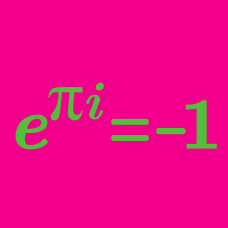Algebra

Complex Numbers - Euler's Formula

Using Euler's formula $e^{ix} = \cos x + i\sin x$, evaluate

$\large e^{i \pi}.$

Which of the following is equivalent to the conjugate of the complex number $4e^{i\pi /4}?$

The complex number $z = -4 + 3i$ can be converted into the polar form $z = re^{i\theta}.$

What is the value of $r?$

If $z = \sqrt{3} + i$, then what is the value of $z^6?$

Hint: You might want to start by converting $\sqrt{3} + i$ into the form $z = re^{i\theta}.$

Given a complex number $z = e^{ix}$, for which of the following values of $x$ is the quantity $\text{Re}(z)\text{Im(z)}$ minimized?

×